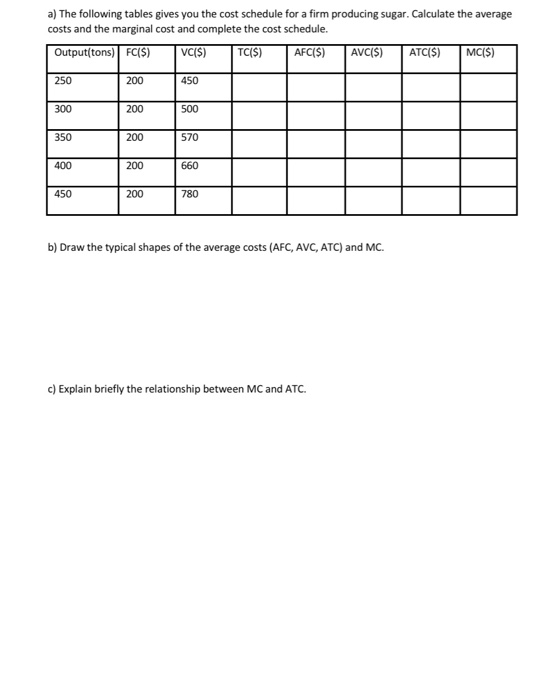### Create an Account

Home / Questions / The following tables gives you the cost schedule fora firm producing sugar Calculate

# The following tables gives you the cost schedule fora firm producing sugar Calculatea) The following tables gives you the cost schedule fora firm producing sugar. Calculate the average costs and the marginal cost and complete the cost schedule Output(tons) FC(S) VCIS) TC(S) AFC(S) AVC(S) ATC(S) MC(S) 450 250 200 300 200 500 350 200 570 400 200 660 450 200 780 b) Draw the typical shapes of the average costs (AFC, AVC, ATC) and MC c) Explain briefly the relationship between MC and ATC.

Feb 06 2020 View more View LessGet Solution